# Q4 20 Points Let (a.) 21 be a sequence of real numbers and a ER such...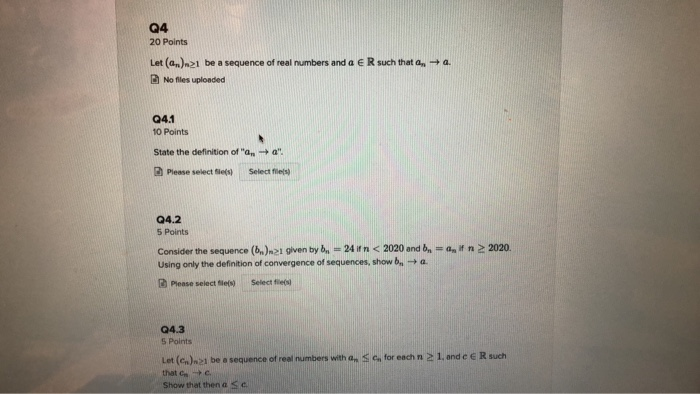Q4 20 Points Let (a.) 21 be a sequence of real numbers and a ER such that .-+ 4. No fles uploaded Q4.1 10 Points State the definition of " a Please select flies a ". Select files Q4.2 5 Points in 2020. Consider the sequence (6.) 1 given by bn = 24 in <2020 and be Using only the definition of convergence of sequences, show b a . Please select file Select file Q4.3 5 Points Let(). be a sequence of real numbers with a se for each n 21. ande e R such that Show that then a SC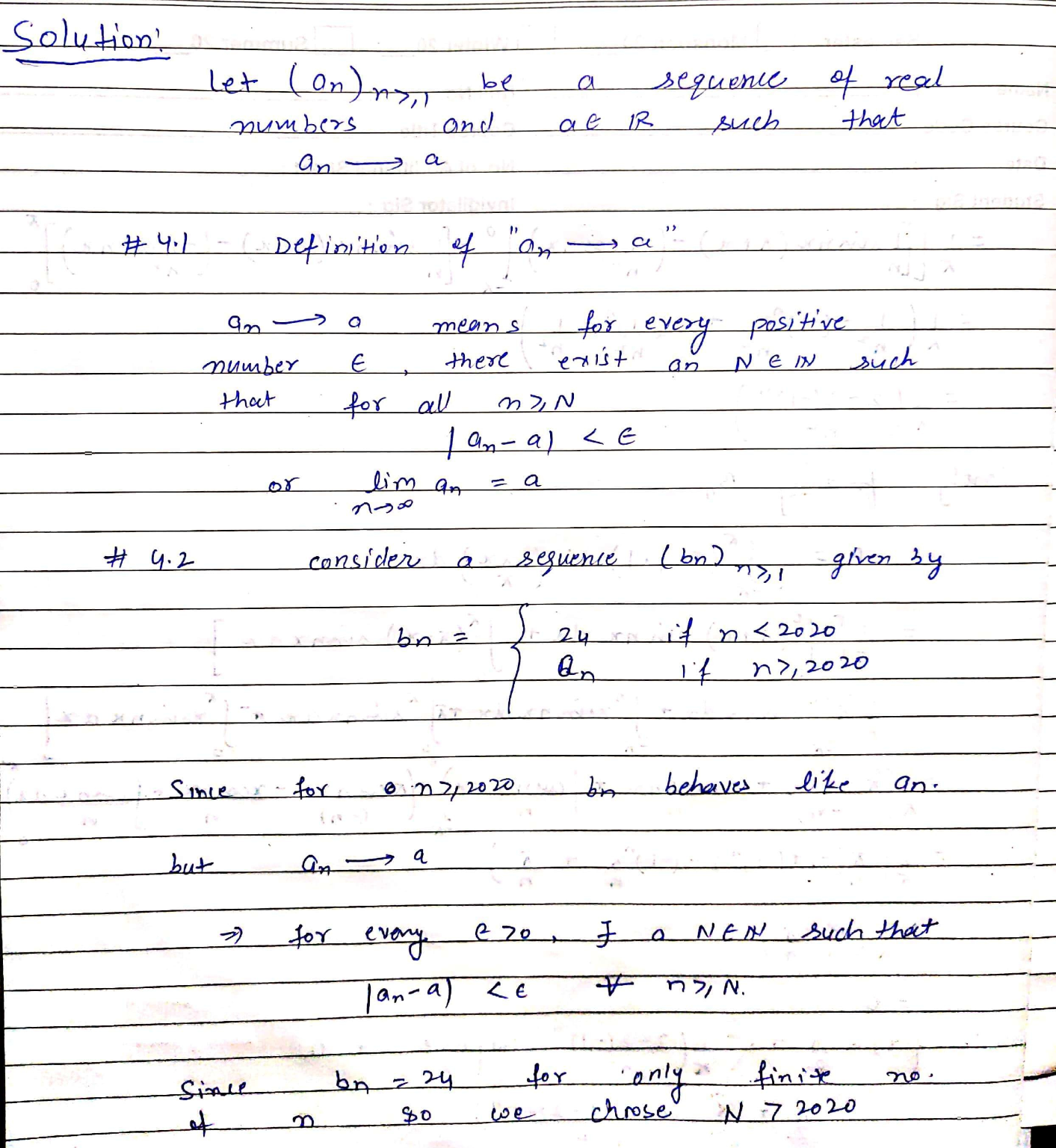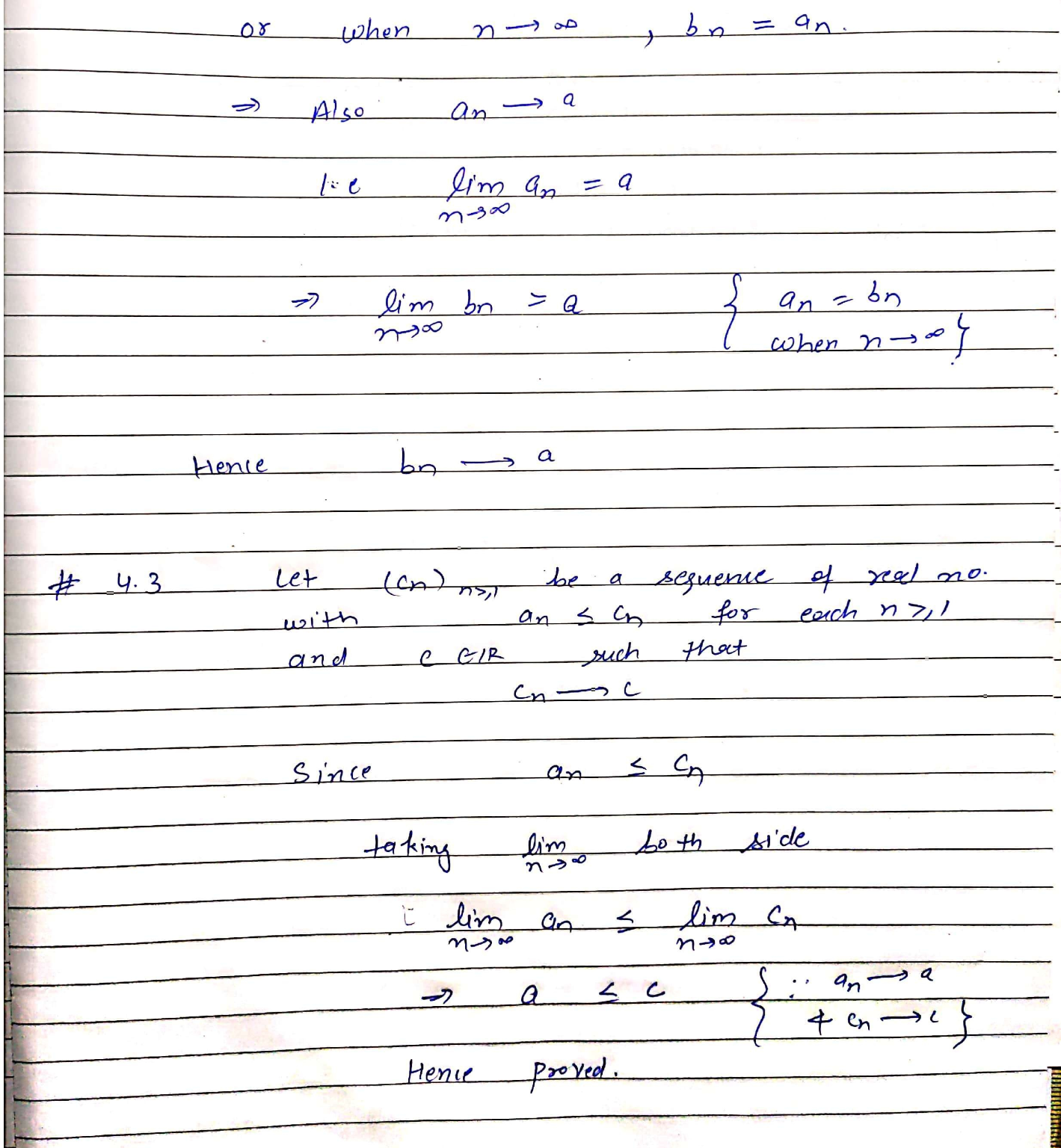#### Earn Coin

Coins can be redeemed for fabulous gifts.

Similar Homework Help Questions
• ### 1. Let {rn;n > 1} be a sequence of real numbers such that rn → x,...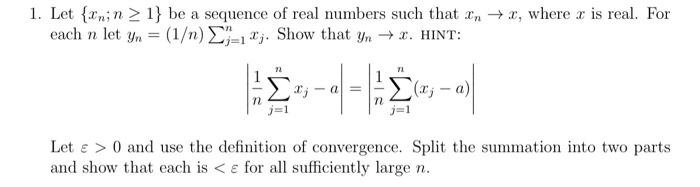1. Let {rn;n > 1} be a sequence of real numbers such that rn → x, where r is real. For each n let yn = (1/n) E*j. Show that yn + x. HINT: (xj – a) Let e >0 and use the definition of convergence. Split the summation into two parts and show that each is < e for all sufficiently large n.

• ### 18. If ai, az, as,... is a bounded sequence of real numbers, define lim sup an...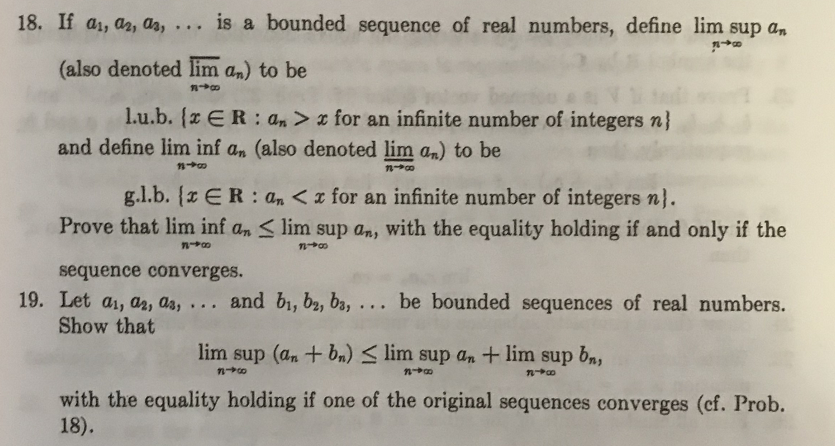18. If ai, az, as,... is a bounded sequence of real numbers, define lim sup an (also denoted lim an) to be --+ n+ l.u.b. {z ER: an > & for an infinite number of integers n} and define lim inf an (also denoted lim an) to be g.l.b. {ER: An <for an infinite number of integers n}. Prove that lim inf an Slim sup an, with the equality holding if and only if the sequence converges. 19. Let ai,...

• ### an+1 for all values of n. What 1. Let {an} be a sequence of positive, real...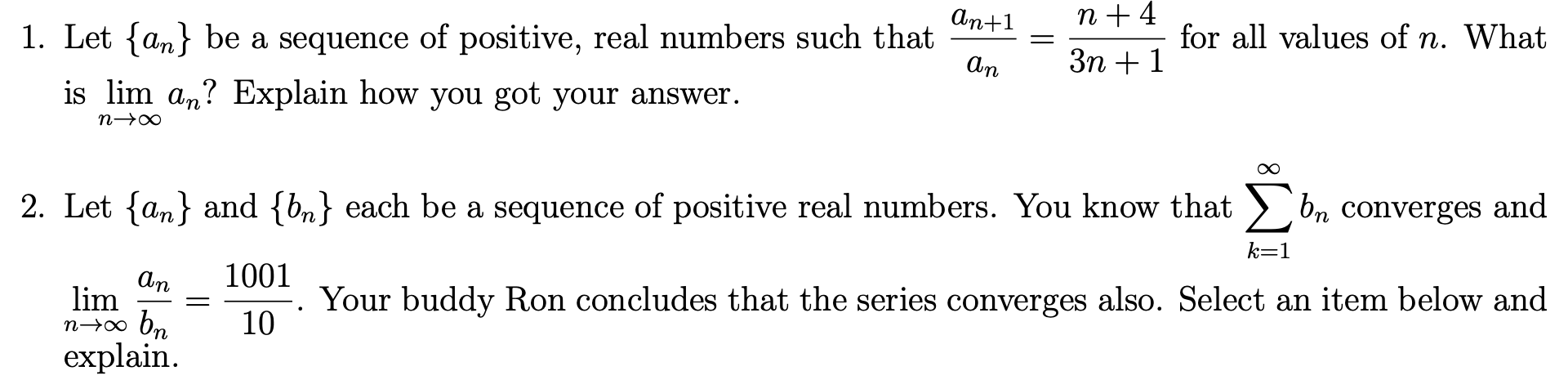an+1 for all values of n. What 1. Let {an} be a sequence of positive, real numbers such that is lim an? Explain how you got your answer. an 3n + 1 n-> 2. Let {an} and {bn} each be a sequence of positive real numbers. You know that ) bn converges and k=1 21. Your buddy Ron concludes that the series converges also. Select an item below and n70 bm 10. explain. lim An _ 1001

• ### please do both question clearly，thank you! Consider the sequence of real numbers 13. 1 1 2'...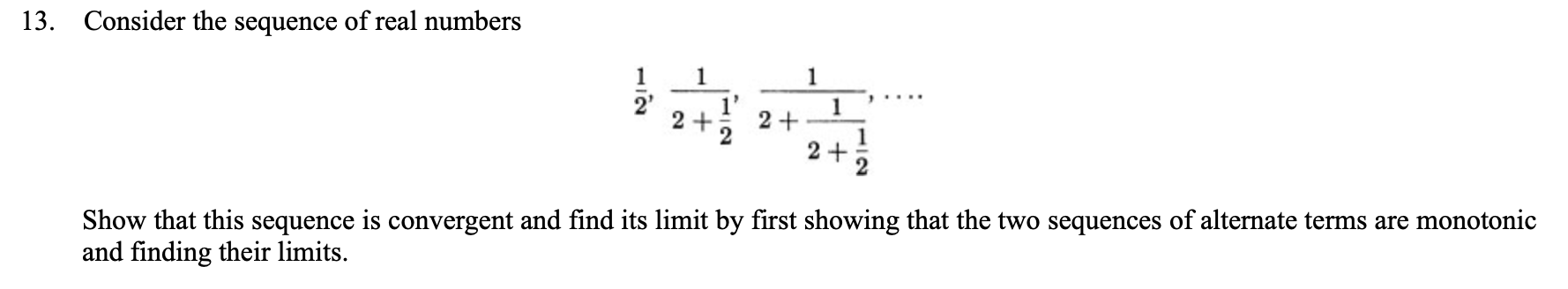please do both question clearly，thank you! Consider the sequence of real numbers 13. 1 1 2' 1 2 + 2 + 1 2 + Show that this sequence is convergent and find its limit by first showing that the two sequences of alternate terms are monotonic and finding their limits. Prove that any sequence in hence we may suppose that each subsequence has a least term.) (Note that this result and the theorem on the convergence of bounded monotonic sequences...

• ### Q4 6 Points Let V be a vector space over R and let Vi, ..., Ur...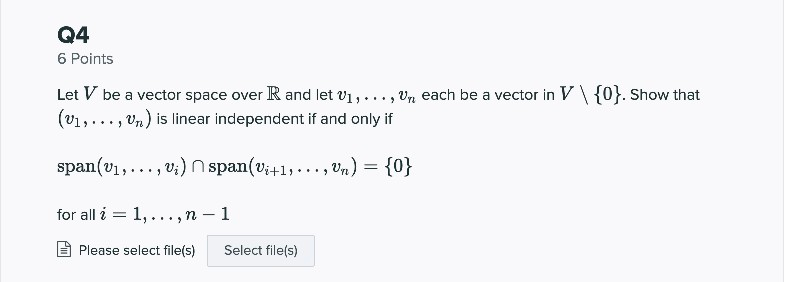Q4 6 Points Let V be a vector space over R and let Vi, ..., Ur each be a vector in V\{0}. Show that (v1,..., Vre) is linear independent if and only if span(v1,..., vi) n span(Vi+1,...,Vn) = {0} for all i = 1,...,n-1 Please select file(s) Select file(s)

• ### Q3 14 Points Consider the vector space P2(R). Let T1, T2, T3 be 3 distinct real...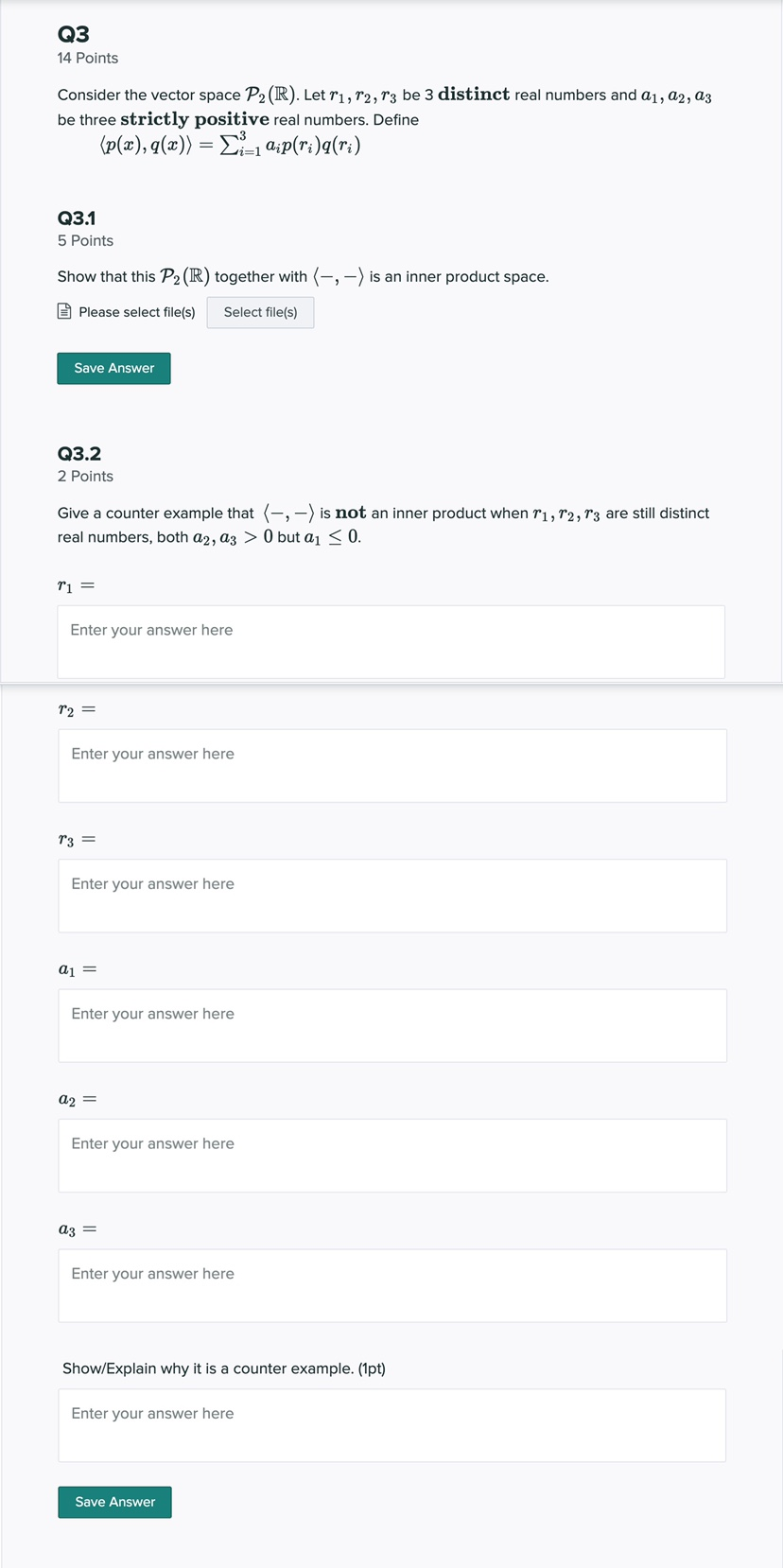Q3 14 Points Consider the vector space P2(R). Let T1, T2, T3 be 3 distinct real numbers and 21, 22, az be three strictly positive real numbers. Define (p(x), q(x)) = Li_1 Qip(ri)q(ri) Q3.1 5 Points Show that this P2 (R) together with (-:-) is an inner product space. Please select file(s) Select file(s) Save Answer Q3.2 2 Points Give a counter example that (-, - ) is not an inner product when T1, 12, 13 are still distinct real...

• ### Q2 15 Points Let n E N and A € Mnxn(R). Define trace(A) = 21=1 Qişi...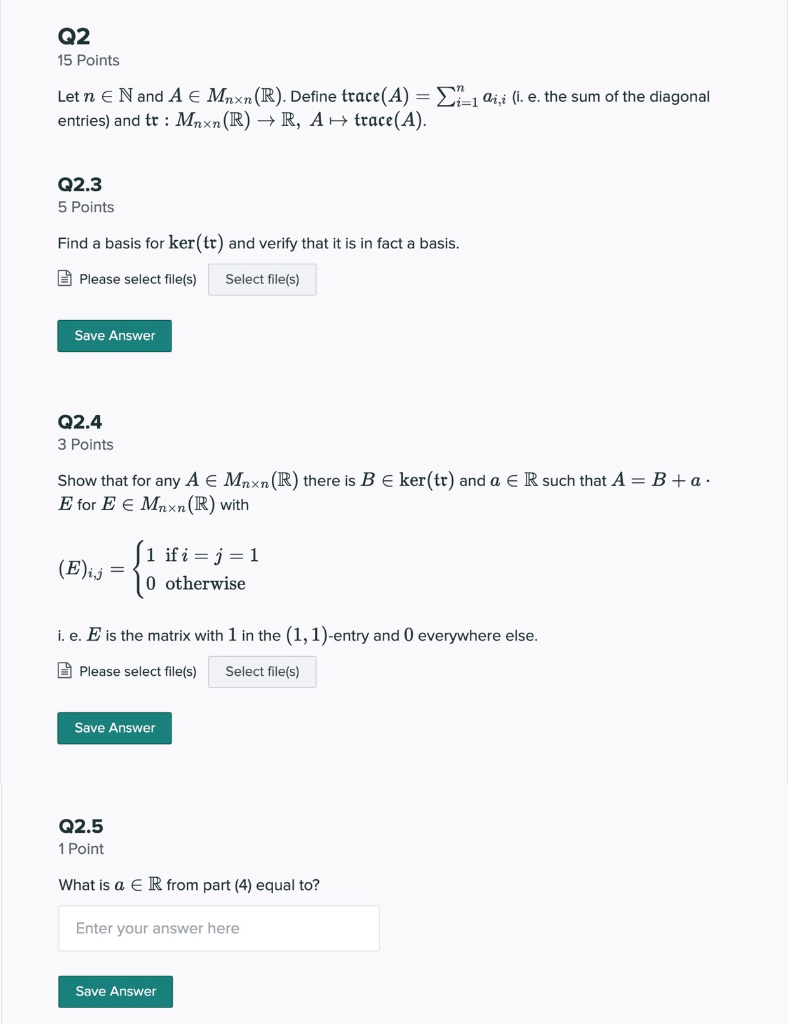Q2 15 Points Let n E N and A € Mnxn(R). Define trace(A) = 21=1 Qişi (i. e. the sum of the diagonal entries) and tr : Mnxn(R) +R, A H trace(A). Q2.3 5 Points Find a basis for ker(tr) and verify that it is in fact a basis. Please select file(s) Select file(s) Save Answer Q2.4 3 Points Show that for any A € Mnxn(R) there is B e ker(tr) and a € R such that A = B+a....